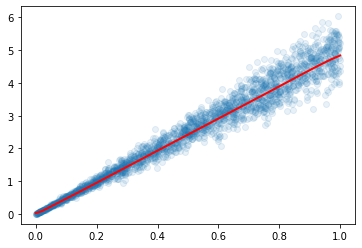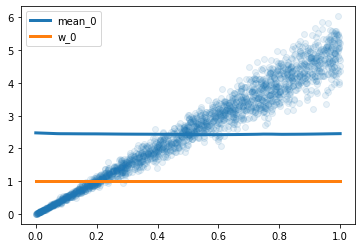# Mixture Density Network

Hello,
I’m trying to get a mixture density network to approximate multivariate distributions. As a pedagogic, toy-example, I’m considering a noisy linear distribution.As a baseline, I’m fitting this with a basic model:
`baseline = nn.Sequential(nn.Linear(1,32), nn.ReLU(), nn.Linear(32,1))`

Which allows me to get:Now, I’m creating a mixture model as such:

``````class MixtureModel(nn.Module):
def __init__(self, k = 5):
super().__init__()
self.base = nn.Sequential(nn.Linear(1,128),
nn.ReLU(),
nn.Linear(128,128),
nn.ReLU())
self.means = nn.Linear(128,k)
self.stds = nn.Linear(128,k)
self.weights = nn.Linear(128,k)
def forward(self, x):
latent = self.base(x)
means = self.means(latent)
stds = F.elu(self.stds(latent)) + 1
w = F.softmax(self.weights(latent), dim = 1)
return means, stds, w
``````

Training goes like this, maximizing log likelihood of observed data:

``````model = MixtureModel()
epochs = 50
losses = []
for epoch in range(epochs):
epoch_loss = []
means, sigma, w = model(xx)
comp = Normal(means, sigma)
mix = Categorical(w)
gmm = MixtureSameFamily(mix, comp)
likelihood = gmm.log_prob(yy)
loss = -likelihood.mean()
loss.backward()
epoch_loss.append(loss.item())
losses.append(np.mean(epoch_loss))
``````

However, after a few iterations of decreasing loss, the performance plateaus and the result are deceiving. Specifically, I was expecting the variance to grow with x, but the network is predicting constants means, weights and variance for all x.Similar behaviours are observed when I increase the number of gaussians.Could someone point me in the right direction ?
Thanks a lot ((:

Mixtures can be hard to identify even with sampling-based methods; even more so with MLE gradient descent methods, that easily get stuck at inferior local optimas.

Usual problems are:

1. mode collapse - as variance parameter (here, sigma) optimization with gradients is tricky/unstable
2. component collapse - as you’re trying to find component weights for untrained components, their training trajectory can be chaotic, and components inferior at initialization are likely to be discarded not trained
3. non-identifiability - if components are not well separated / are overlapping, optimizers can get confused

In your specific case, I don’t think you can discover 5 clusters training on scalar inputs without inherent (x,y) clusters. Check if it trains (finds slope) with k=1, if not - decrease learning rate or constrain sigma (fix to 1 for a start).

Hi @Mehdi I am doing a similar exercise, but I want to ask you. Suppose you solved your issue. Then, how would you predict in inference given that you have just 1 real y target y but your NN predicts k-number of y’s?